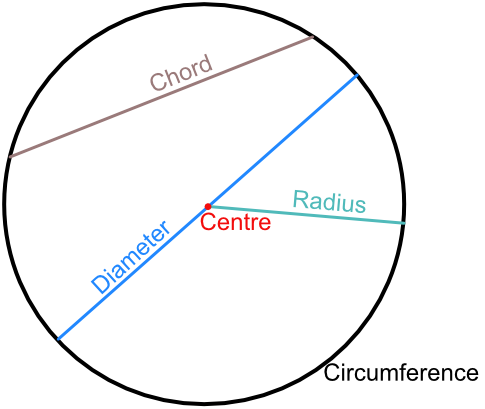Properties of Circles

## Properties of Circles

A circle is a line drawn at a fixed distance from a point. The point is called the centre, and the line that is drawn a fixed distance from it is called the circumference.

The distance from the centre to the circumference is the radius.

A line joining one part of the circumference to another part of the circumference is known as a chord. A chord that goes through the centre of a circle is a diameter, and has twice the length of the radius.## Example 1

A circle has a radius of 8cm. What is the longest chord that can be drawn on the circle?

The longest chord must go through the centre of the circle. This is the diameter; the diameter is twice the value of the radius. 2 x 8 = 16cm.